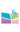﻿2020.01.06，范恩贵教授，复旦大学，Inverse scattering transformation for Fokas-Lenells equation with non-zero boundary conditions -中国矿业大学（北京）理学院网站2020.01.06，范恩贵教授，复旦大学，Inverse scattering transformation for Fokas-Lenells equation with non-zero boundary conditions

: 范恩贵教授  复旦大学

要：In this talk, we focus on the inverse scattering transformation  for the Fokas-Lenells equation with nonzero boundary conditions via the Riemann-Hilbert approach. Based on the Lax pair of the FL equation, the analyticity and symmetry, asymptotic behavior of Jost solutions and scattering matrix are discussed in detail. We further present a generalized RH problem associated with the FL equation, from which a reconstruction formula between potential and the Riemann-Hilbert problem is obtained.  The N-soliton solutions of the FL equation  is obtained via solving the RH problem.

间： 202016 8:15---9:00

点： 逸夫楼1537